# Unit 5: Account Current Notes | Study Principles and Practice of Accounting - CA Foundation

## CA Foundation: Unit 5: Account Current Notes | Study Principles and Practice of Accounting - CA Foundation

The document Unit 5: Account Current Notes | Study Principles and Practice of Accounting - CA Foundation is a part of the CA Foundation Course Principles and Practice of Accounting.
All you need of CA Foundation at this link: CA Foundation

5.1 Introduction
An Account Current is a running statement of transactions between parties for a given period of time and includes interest allowed or charged on various items. It takes the form of an ledger account.
Some of the situations when account current is prepared are:
1. It is prepared when frequent transactions regularly take place between two parties. An example is of a manufacturer who sells goods frequently to a merchant on credit and receives payments from him in instalments at different intervals and charges interest on the amount which remains outstanding.
2. A consignee of goods can also prepare an Account Current, if the latter is to settle the account at the end of the consignment & interest is chargeable on outstanding balance.
3. An Account Current also is frequently prepared to set out the transactions taking place between a banker and his customer.
4. It is prepared when two or more persons are in joint venture and each co-venture is entitled to interest on their investment. Also, no separate set of book is maintained for it.
An Account Current has two parties - one who renders the account and the other to whom the account is rendered. This is indicated in the heading of an Account Current, which is like the following: "A in Account Current with B". It implies that A is the customer, and the account is being rendered to him by B.

5.2 Preparation of Account Current
There are three ways of preparing an Account Current:
(i) With help of interest table
(ii) By means of products
(iii) By means of products of balances

5.2.1 Method 1: Preparation of Account Current with the help of Interest Tables-Individual Method
According to this method, all the transactions are arranged in the form of an account. There are two additional columns on both the sides of such an account.
(a) One column is meant to indicate the number of days counted from the due date of each transaction to the date of rendering the account. If no specific date is mentioned as the date on which payment is due, the date of the transactions is presumed to be the due date.
(b) The other column is meant for writing interest. With the help of ready made tables, interest due on different amounts at given rates for different periods of time is found out and this is entered against each item separately.
The interest columns of both the sides are totalled up and the balance is drawn.

ILLUSTRATION 1
Prepare Account Current for Nath Brothers in respect of the following transactions with Shyam: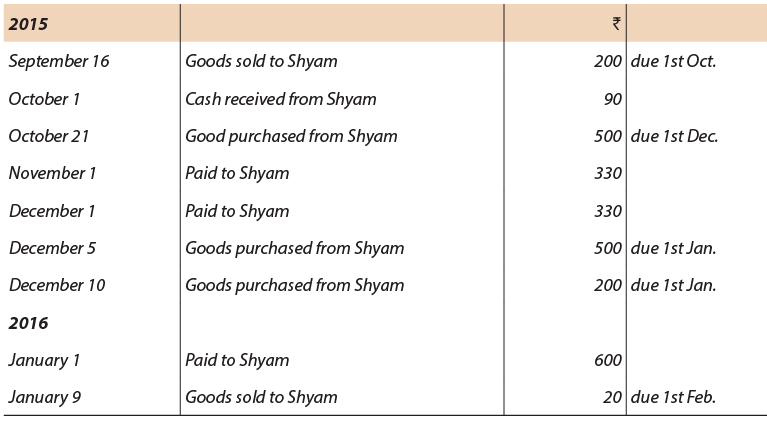The account is to be prepared upto 1st February. Calculate interest @ 6% per annum. (1 year = 365 days)
Solution:
Shyam in Account Current with Nath Brothers (Interest to 1st February, 2016 @ 6% p.a.)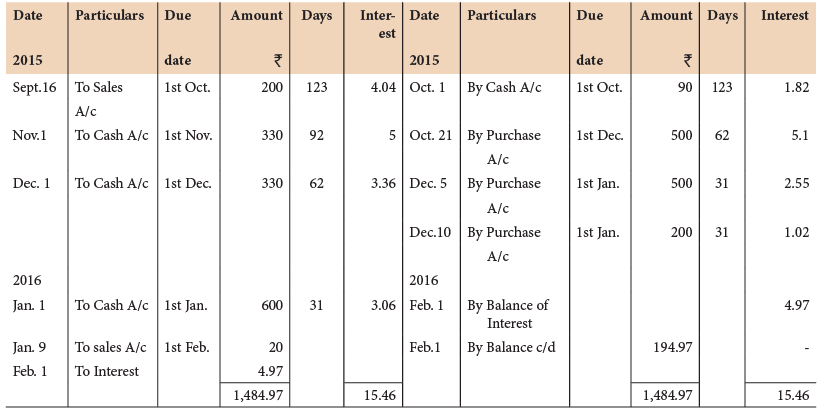Tutorial Notes:
(1) While counting the number of days, the date of due date is ignored and the date upto which the account is prepared, is included.
(2) While counting the number of days, for opening balances, the opening date as well as date upto which the account is prepared, is counted.
Calculation of days: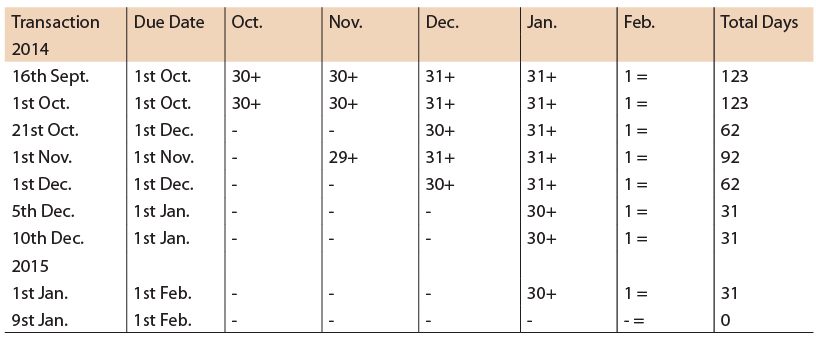5.2.2 Method 2: Preparation of Account Current by means of Products; Product Method
When this method is followed, the way of preparing the Account Current remains the same. In this method is only the method of calculating interest is different.
Under the previous method, interest columns are provided on both the sides of the Account Current, and interest in respect of each item is found out from the ready-made interest tables. In this method, interest columns are replaced by "product" columns. Product in this case is the amount multiplied by the number of days for which it has been outstanding. Interest on a certain sum of money for a certain number of days is the same thing as interest on the product for one day. In other words, with a view to reduce the period of each transaction to one day, the amount of each transaction is multiplied by the number of days. This product is entered against each transaction the product column.

The remaining steps are as follows:
(a) Find out the balance of the products on the two sides.
(b) Calculate interest at the given rate on the balance of the products for a single day.
(c) Enter interest on the appropriate side in the amount column. This entry is made on the side other than that on which the balance of products appears.
Taking Illustration 1 Account Current by means of Product is explained below :
Shyam in Account Current with Nath Brothers (Interest to 1st February, 2016 @ 6% p.a.)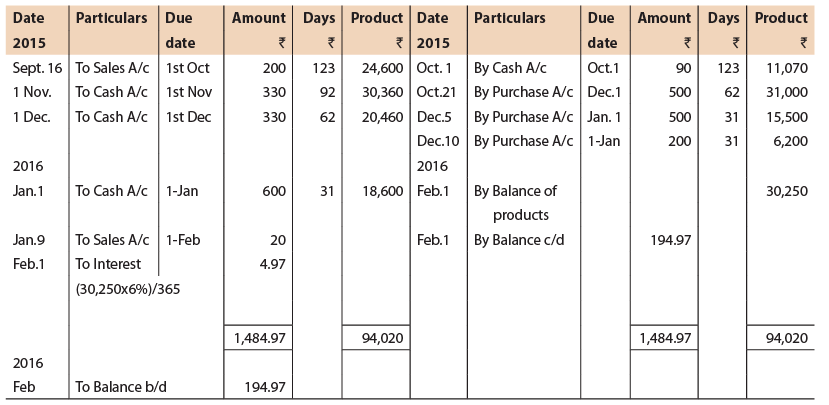5.2.3 Method of Computing the numbers of Days
Usually any of the following two methods is used for calculating the number of days.
1. Forward Method- Under this method the number of days are calculated from the due date of the transaction to the date of closing the account.
2. Backward (or Epoque Method)- Under this method, the number of the days are calculated from the opening date of statement to the due date of transaction.
Example
From the following particulars, make up an Account Current to be rendered by Mr. X to Mr. Y on 31st December, 2016 taking interest into account at the rate of 18% p.a.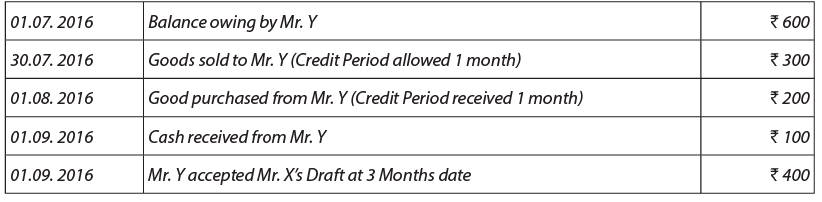You are required to prepare the Account Current according to interest on individual transaction under the Forward and Backward methods.
Solution:
(a) Product of individual Transaction Method (Forward Method)
Mr. Y in Account Current with Mr. X (interest to 31st Dec. 2016 @ 18% p.a.)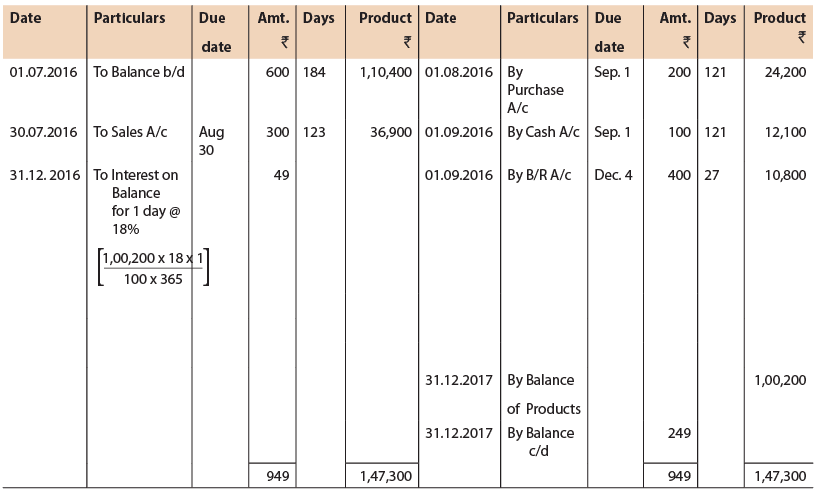(b) Product of individual Transaction Method (Epoque Method)
Mr. Y in Account Current with Mr. X (interest to 31st Dec. 2016 @ 18% p.a.)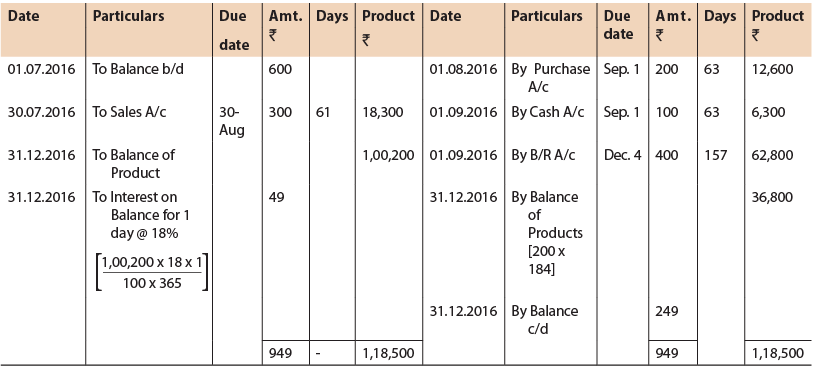ILLUSTRATION 2
From the following particulars prepare the account current to be rendered by Mr. Singh to Mr. Paul as on 31st August, 2016. Interest must be calculated @ 10% p.a. (1 year = 365 days)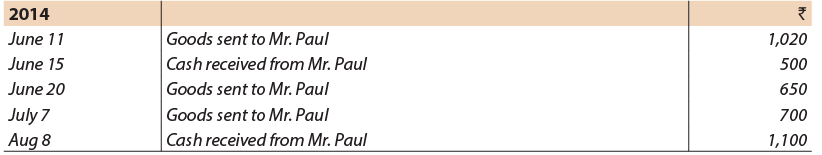Solution: Mr. Paul in Account Current with Mr. Singh (Interest to 31st August, 2016 @ 10% p.a.)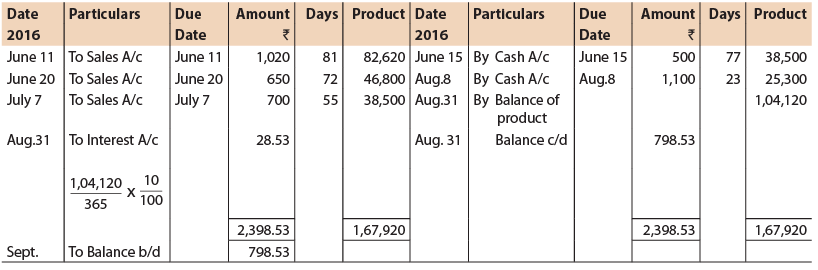ILLUSTRATION 3
From the following particulars make up an Account Current to be rendered by S. Dasgupta to A. Halder at 31st Dec. reckoning interest at 5% p.a. (assume 1 year = 365 days)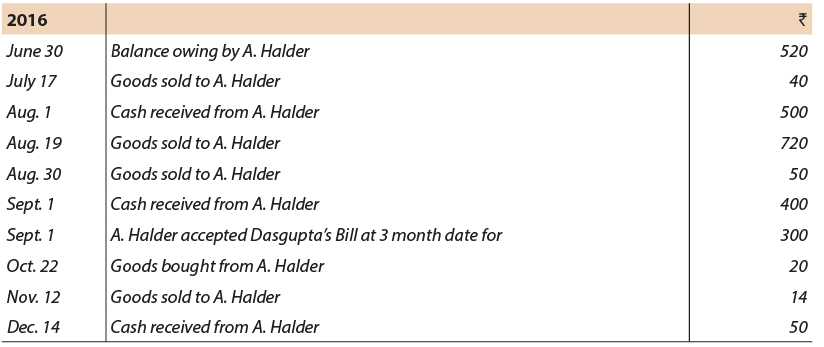Solution:
A. Halder in Current Account with Mr. S. Dasgupta (Interest to 31st December, 2016 @ 5% p.a.)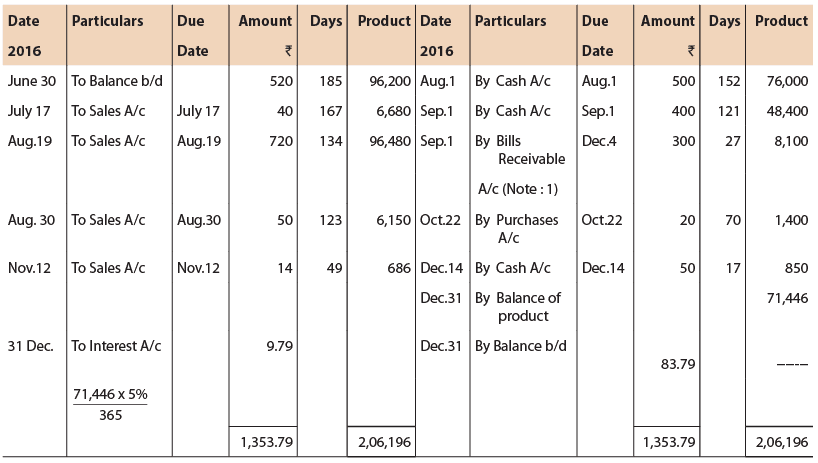Note: It is assumed that the bill was honoured on due date. The due date of the bill should be treated as date of payment and days to be calculated from the due date of account.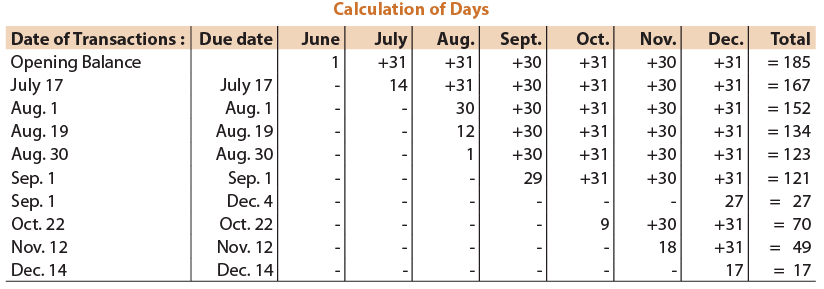Note: While counting the number of days, for opening balances, the opening date as well as date upto which the account is prepared, is counted.

ILLUSTRATION 4
From the following prepare an account current, as sent by A to B on 30th June, 2016 by means of products method charging interest @ 6% p.a: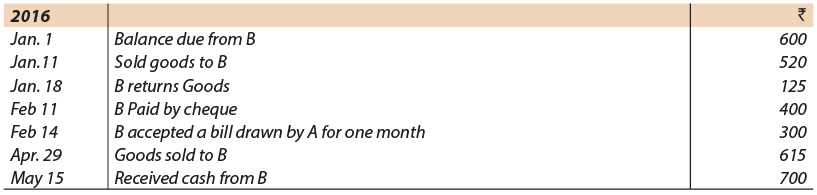Solution:
B in Account Current with A for the period ending on 30th June, 2016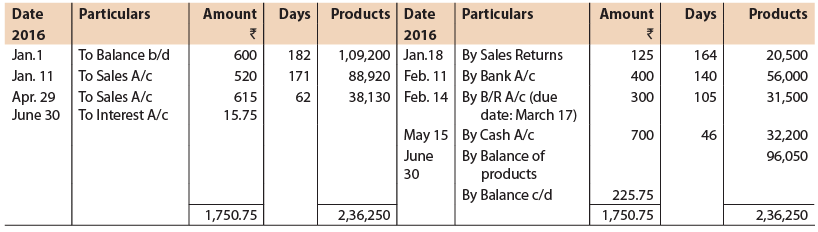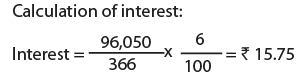Red - Ink Interest: In case the due date of a bill falls after the date of closing the account, then no interest is allowed for that. However, interest from the date of closing to such due date is written in "Red-Ink" in the appropriate side of the 'Account current'. This interest is called Red-Ink interest. This Red Ink interest is treated as negative interest. In actual practice, however the product of such bill [value of bill X (due date-closing date) is written in ordinary ink in the opposite side on which the bill is entered]. It means interest from future date from date of account current i.e., present date. In earlier periods, it was written in red ink; hence it got the name of red ink interest. It implies that rebate will be allowed on interest paid/ received, if settlement of future due transaction is done on account current date
This can also be understood in a different way. In an account current, interest is calculated on the amount of a bill from the date of transaction to the closing date of the period concerned. In case the due date of the bill falls after the closing date of the accounts, then no interest is allowed for that period. Such interest is customarily written in red ink in the appropriate side of the account current. The interest is called Red-Ink interest and is treated as negative interest.

ILLUSTRATION 5
Following transaction took place between X and Y during the month of April, 2016.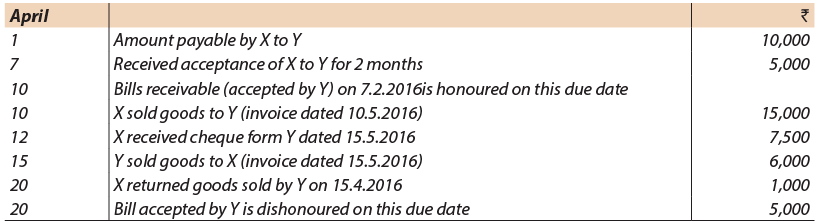You are required to make out an account current by products method to be rendered by X to Y as on 30.4.2016, taking interest into account @ 10% p.a. (assume 1 year = 365 days)
Solution:
‘Y’ In Account Current with ‘X’ (Interest to 30th April, 2016 @ 10% p.a.)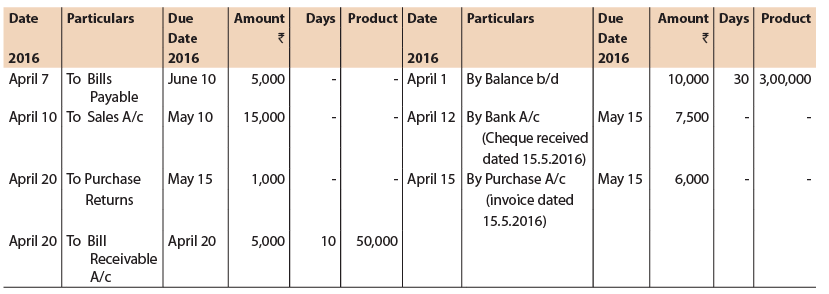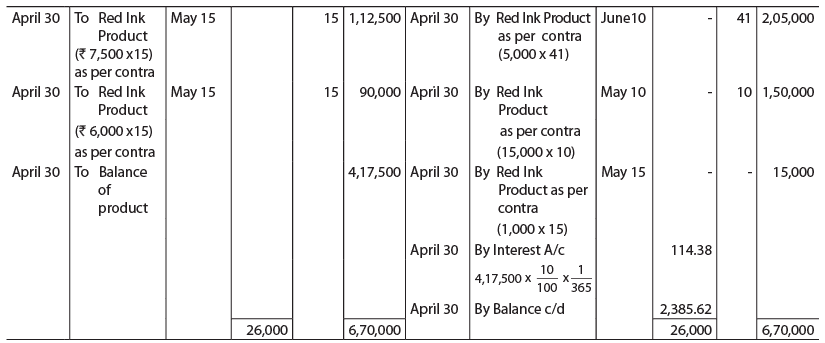No entry is required for matured bill on 10th April since party is not contracted.

5.2.4 Method 3: Preparation of Account Current by Means of Product of Balances in case of Banks.
This method, also known as periodic balance method, is usually adopted in the case of banks where the balance of account is taken out after every transaction. In this case, the number of days written against each transaction are the days counted from its date or due date to the date of the following transaction. In the case of the last transaction, the number of days is counted to the close of the period.
Each amount is multiplied with the number of days. If the amount represents a debit balance, the product is entered in the Dr. Product column; and if it represents a credit balance, the product is written in the Cr. Product column. The Dr. Product and Cr. Product columns are then totalled up. Interest is calculated on each total at the given rate of interest; and the net interest is ascertained. If net interest is payable to the customer, it will appear as “By Interest A/c”, and if it is due from the customer, it will appear as “To Interest A/c”.

ILLUSTRATION 6
On 2nd January, 2016 Vinod opened a current account with the Allahabad Bank Limited; and deposited a sum of ₹ 30,000.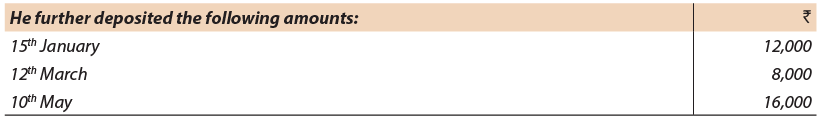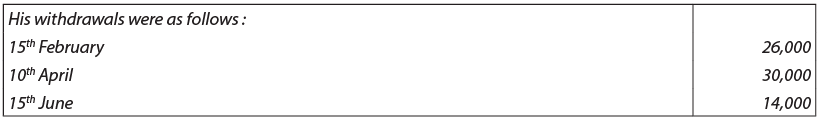Show Vinod’s a/c in the ledger of the Allahabad Bank. Interest is to be calculated at 5% on the debit balance and 2% on credit balance. The account to be prepared as on 30th June, 2016. Calculation may be made correct to the nearest rupee. (assume 1 year = 365 days)
Solution:
Vinod Current Account with Allahabad Bank Ltd.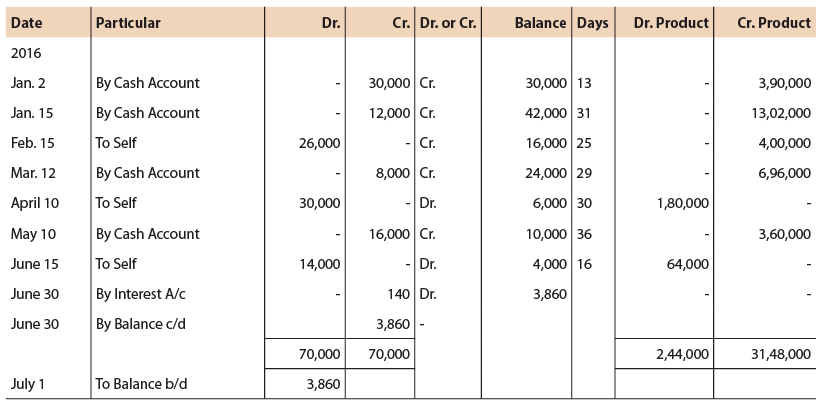* Interest is calculated as follows:
On ₹ 31,48,000 @ 2% for 1 day = ₹ 172.49
On ₹ 2,44,000 @ 5% for 1 day = ₹ 33.42
Net Interest = ₹ 139.07 (₹ 172.49- ₹ 33.42)

The document Unit 5: Account Current Notes | Study Principles and Practice of Accounting - CA Foundation is a part of the CA Foundation Course Principles and Practice of Accounting.
All you need of CA Foundation at this link: CA FoundationUse Code STAYHOME200 and get INR 200 additional OFF

## Principles and Practice of Accounting

44 videos|213 docs|82 tests

Track your progress, build streaks, highlight & save important lessons and more!

,

,

,

,

,

,

,

,

,

,

,

,

,

,

,

,

,

,

,

,

,

;